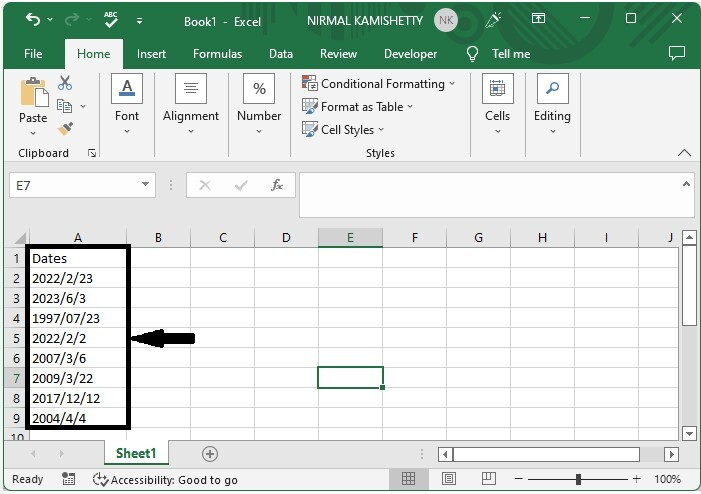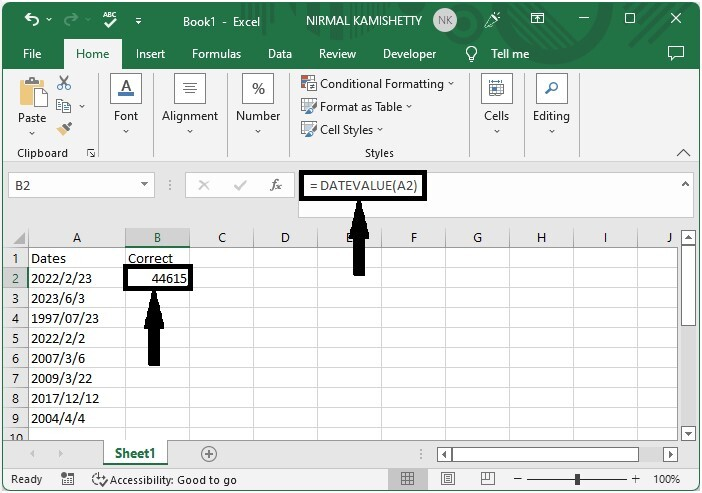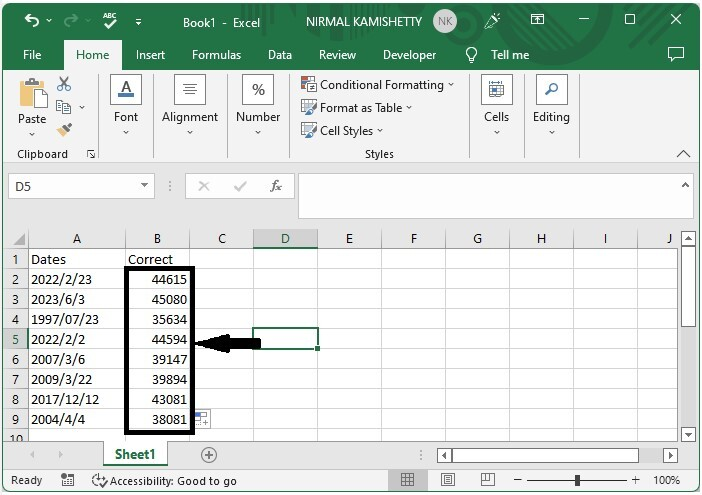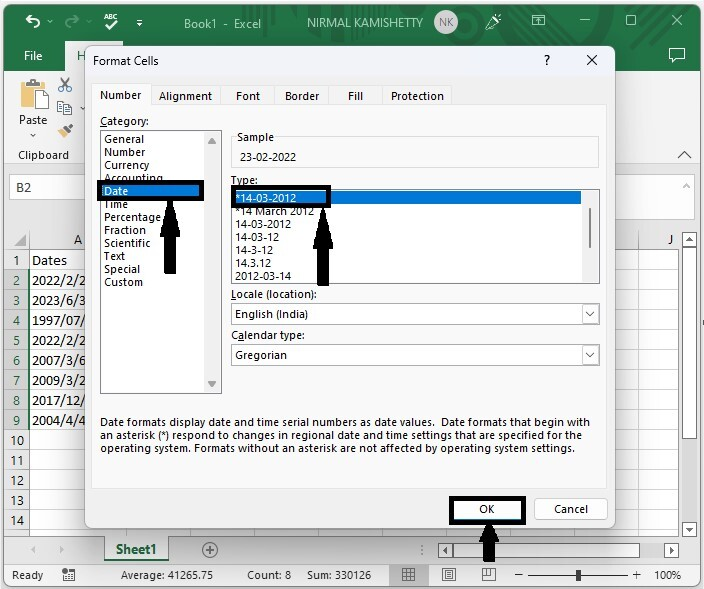# How to Convert Various Non-Standard Date Formats to Standard Date in Excel?

Sometimes you may have observed that the dates in the sheet are not in a valid format. If the dates are not valid, we will not be able to perform any operations. So, it is very important for us to know different ways in which we can convert them into a valid format. Read this tutorial to learn how you can convert various non‑standard formats to standard formats in Excel. We can solve this task by using formulas and formatting cells in Excel.

## Converting Non-Standard Date Formats to Standard Date in Excel

Here we will use the DATEVALVE formula to get any one of the values, then use the auto-fill handle to get all the results, then use the format function to get the final result. Let's take a look at a simple procedure for converting nonstandard date formats to standard dates in Excel.

### Step 1

Let us consider an Excel sheet where the dates in the sheet are not in a valid format, as shown in the below image.Now, in our case, click on an empty cell, B2, and enter the formula = DATEVALUE(A2) and Click Enter to get our first result. A2 is the cell address in the formula.### Step 2

To get all the values, drag down from the first result using the auto-fill handle, and it will look similar to the below image.Now we need to convert them to dates.

### Step 3

To convert them into the date format, Select the values, then right-click and select format cells; in the pop-up window, click on date and click OK.

Select data > Right click > Date > OKNote − If the invalid format includes the month name, such as 2017-Apr-12, we can use the formula =DATEVALUE(RIGHT(A1,2)&"-"&MID(A1,6,3)&"-"&LEFT(A1,4).

## Conclusion

In this tutorial, we used a simple example to demonstrate how you can convert nonstandard date formats to normal formats in Excel.

Updated on: 07-Mar-2023

904 Views